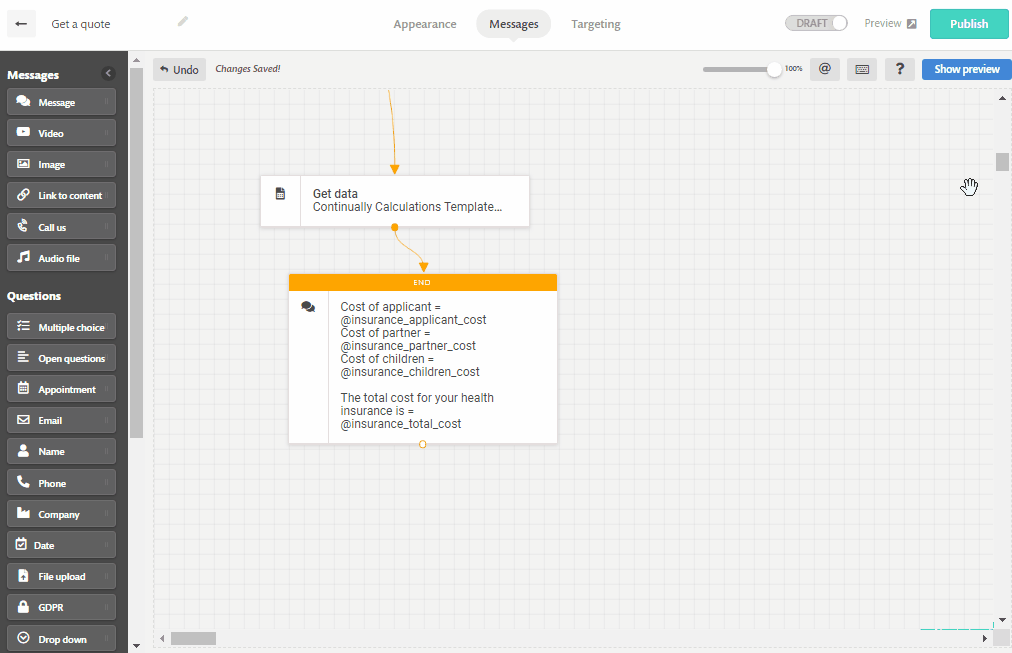# How to make calculations using Google Sheets

To do calculation we need to pass the lead's info or answers to google sheets, make the calculations at Google Sheets then get the data back to Continually and present it to the lead or create different paths depending on the value.

Note: This is an advanced tutorial that requires knowledge of Google Sheets functions.

#### Possible use cases

• To sum multiple custom fields and get the result.
• To make a quiz and add the result.
• To make different paths for different ranges.
• Multiplying or dividing numbers.
• Get a percentage.
• Calculate a formula.

## Step 1.  Create the bot

In this tutorial we will explore a use case where a user will need to provide data to get a quote for a health insurance. We will then use this data to do calculations and get a quote.

For the sake of simplicity, we will use a simple equation and pricing terms.

Health Insurance Quote = Cost of the applicant depending on their age + cost of  partner +  Cost of children

Now let's get to the Continually part. Create a new bot and add questions to capture the, Name, Email, Phone, their age, whether they want to add their partner or not, how many children they want to include and whether they want to include their parents. You will save each of these questions into custom fields so we can send them later to Google sheets.

It will look like this :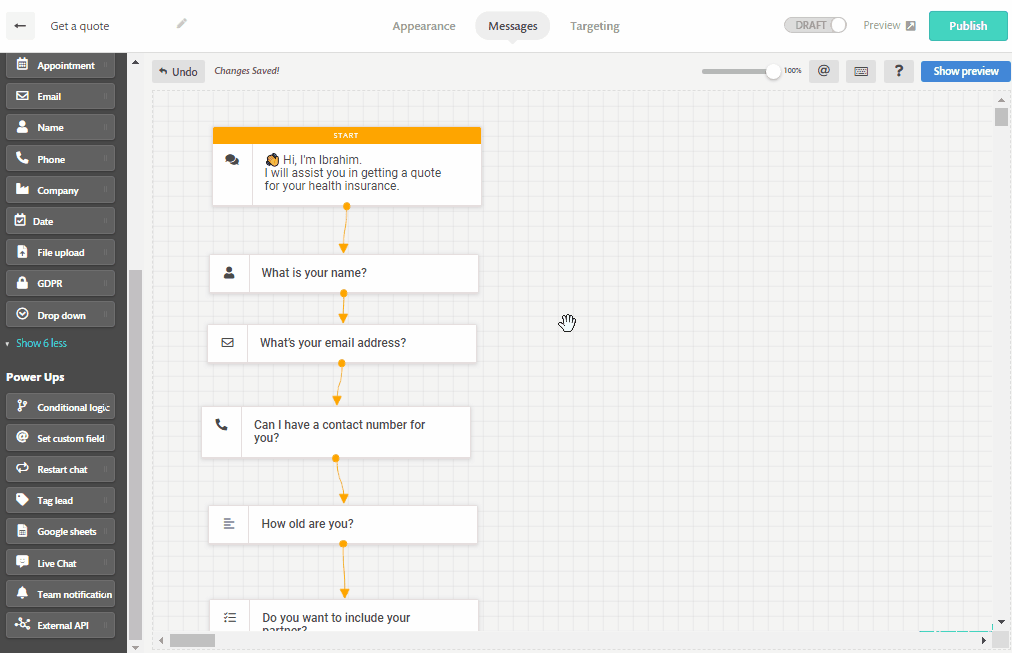### Step 2. Send data to Google Sheets

Now for the Google sheet part, we created a template that you can use to make these calculations. The sheet consists of the basic info like Name, Email, Phone, Company, along with some columns for custom fields and some columns for calculations. You can increase the number of columns for calculations or custom fields as you want.

Open the template from this link, create a copy of it and add it to your drive. Make the necessary edits to the template and add a Google sheet object in the bot builder. Choose the "Add New Row" option and start mapping the columns with their value.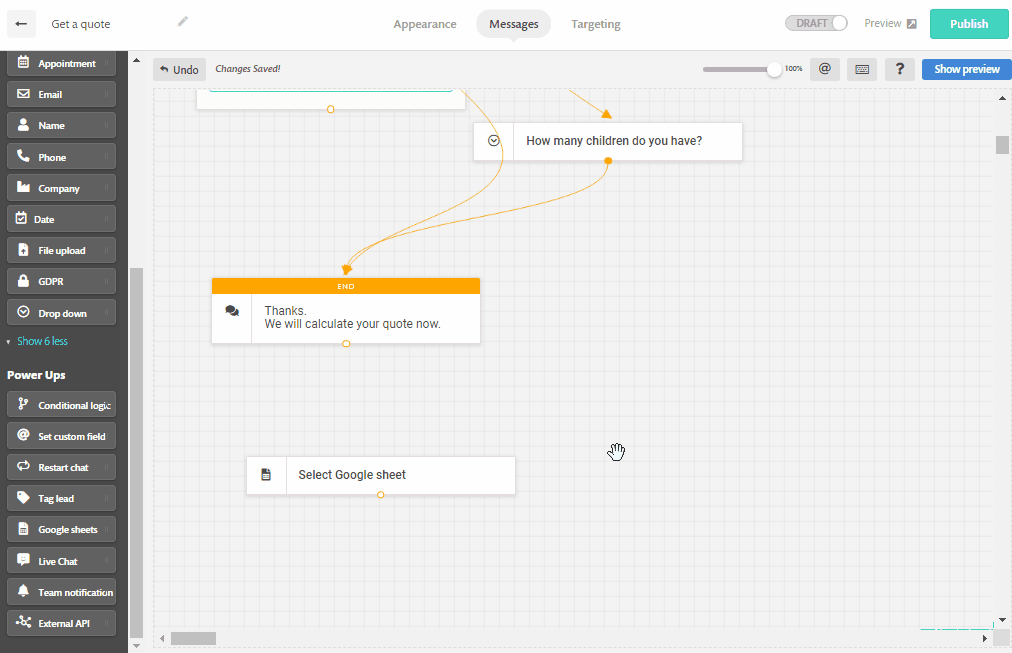Let's test it in Preview.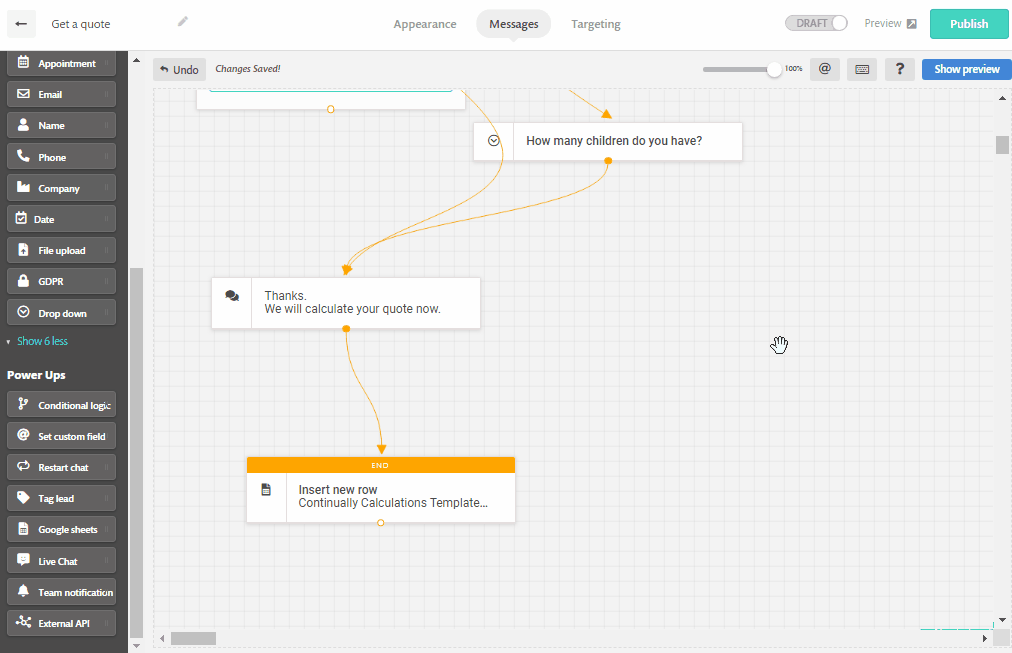Everything looks to be working correctly.

## Step 3. Do calculations

We will do this in two parts. First we will calculate the cost for each person then we will sum the total cost.
Let's say the cost for the applicant or their partner will be = (Age+150)*2.3

The cost for the children will be = No. of Children * 100

To make the formula apply for all new rows, we will use the Arrayformula function, this function will be inserted in the first row of the data and it will carry the calculations for the rest of the column.

In this example we have the Age of applicant in the column E, age of partner in column F, the No. of children in column G. The formula that calculates the cost of the applicant will be :  =ARRAYFORMULA(IF(E2:E="","",(E2:E+150)*2.3))

Formula breakdown:

`=ARRAYFORMULA(` : this is the function responsible for executing the calculations on all rows.

` IF(E2:E="","", ` : The if function is used to check if the cell is empty, then do nothing. if it is not empty then do the calculation. We added the range as E2:E to make the function start from the second row to the end of the column.

` (E2:E+150)*2.3)) ` : This is the calculation that will be carried when the cell is not empty.

The formula for partner's cost will be : =ARRAYFORMULA(IF(F2:F="","",(F2:F+150)*2.3))

The formula for the cost of children will be =ARRAYFORMULA(IF(G2:G="","",(G2:G*100)))

Now we need to do one more calculation to sum the result of these 3 formulas, so in a cell next to them we will add the summation formula : =ARRAYFORMULA(IF(K2:K="","",(K2:K+L2:L+M2:M)))

The cost of the applicant exists in column K, the cost of partner is in column L and the cost of children's insurance is in column M.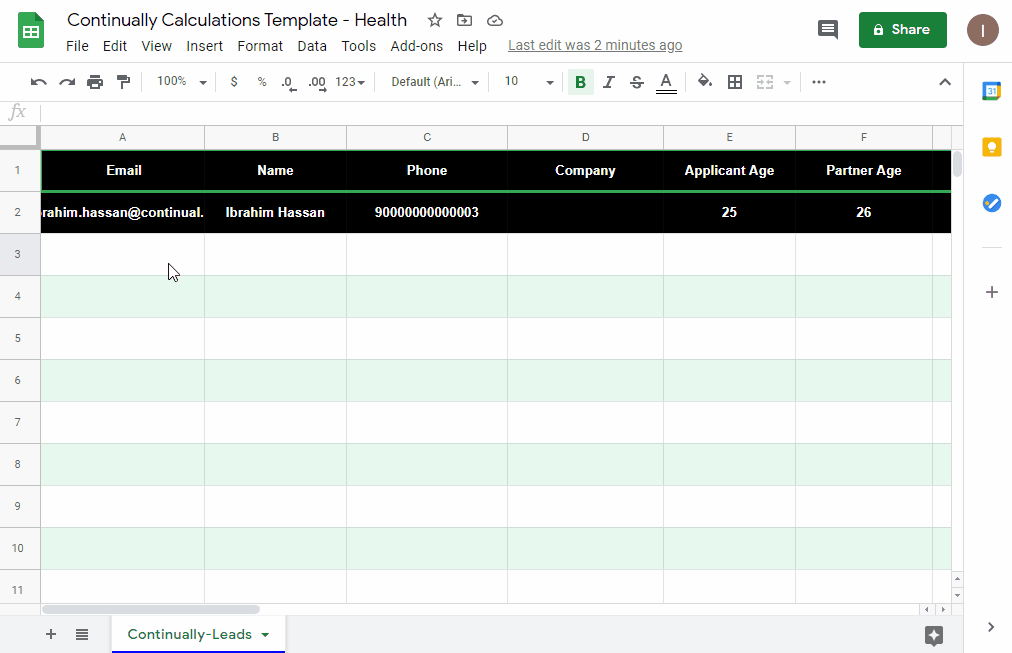## Step 4. Retrieve results

This is the simplest step, just add another Google Sheet object and choose the action Get Data. In the Lookup column, choose Email. We will use the email as the identifier for the customer, you can combine more than one field to get a more complex identifier if you think your customer will use this bot with the same email multiple times.

Get data from the calculations columns and save them into custom fields.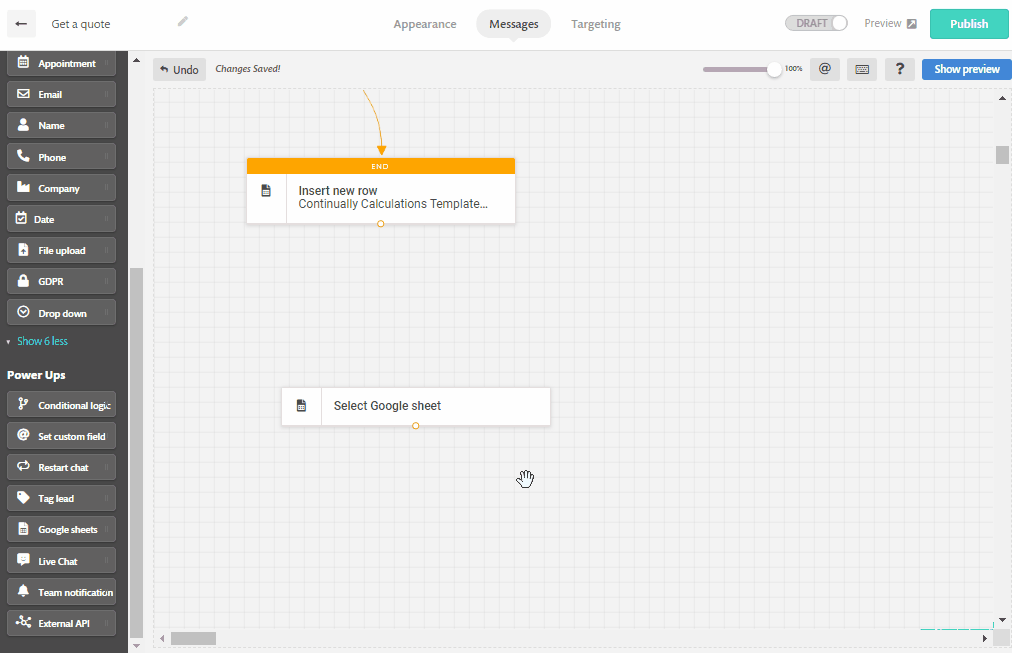Add message and use the cost custom fields to show the quote to the lead.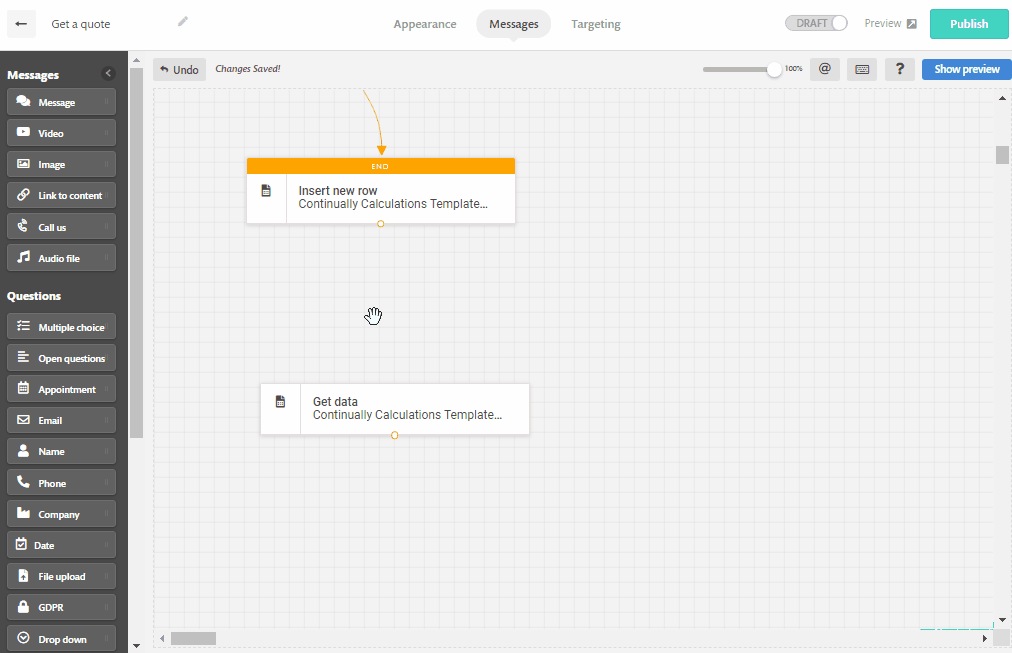Now let's test it.